# Coordinate Geometry-NCERT Solutions

Class X Math
NCERT Solution for Coordinate Geometry
Exercise 7.1
Q.1.   Find the distance between the following pairs of points:
(i) (2, 3), (4, 1) (ii) (–5, 7), (–1, 3) (iii) (a, b), (–a, –b)
Sol. (i) Here x1 = 2, y1 = 3, x2 = 4 and y2 = 1
∴ The required distance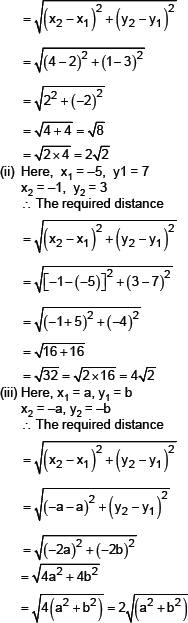Q.2.   Find the distance between the point (0, 0) and (36, 15). Can you now find the distance between the two towns A and B discussed in Section 7.2 of the NCERT textbook?
Sol. Part-I
Let the points be P(0, 0) and Q(36, 15).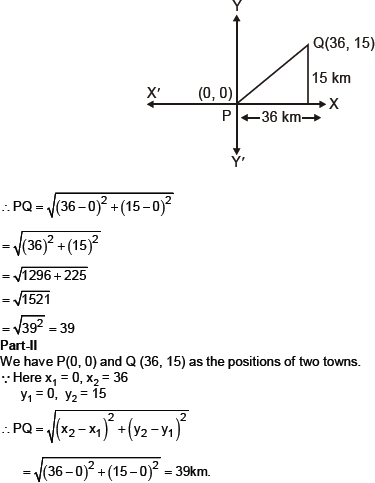Q.3.   Determine if the points (1, 5), (2, 3) and (�2, �11) are collinear.
Sol. Let the points be A(1, 5), B (2, 3) and (�2, �11)
A, B and C are collinear, if
AB + BC = AC
AC + CB = AB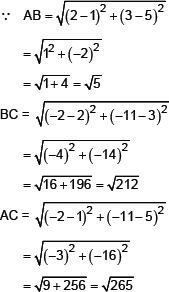But AB + BC ≠ AC
AC + CD ≠ AB
BA + AC ≠ BC
∴ A, B and C are not collinear.
Q.4.   Check whether (5, –2), (6, 4) and (7, –2) are the vertices of an isosceles triangle.
Sol. Let the points be A (5, –2), B (6, 4) and (7, –2).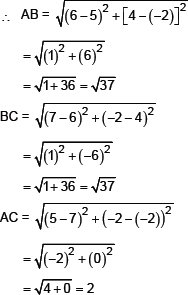We have AB = BC ≠ AC
∴ΔABC is an isosceles triangle.
Q.5.   In a classroom, 4 friends are seated at the points A, B, C and D as shown in Fig. Champa and Chaneli walk into the class and after observing for a few minutes Champa asks Chaneli, “Don�t you think ABCD is a squar?” Chaneli disagrees. Using distance single formula, find which of them is correct.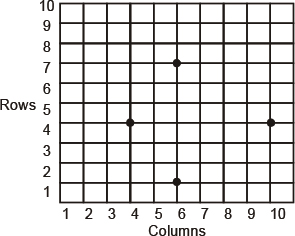Sol. Let the number of horizontal columns represent the x-coordinates whereas the vertical rows represent the y-coordinates.
∴ The points are:
A(3, 4), B(6, 7), C(9, 4) and D(6, 1)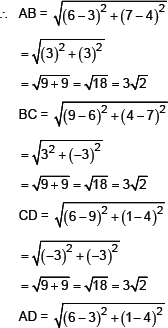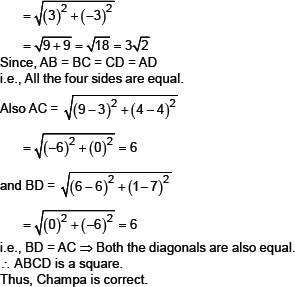Q.6.   Name the type of quadrilateral formed, if any, by the following points, and give reasons for your answer:
(i) (�1, �2), (1, 0), (�1, 2), (�3, 0)
(ii) (�3, 5), (3, 1), (0, 3), (�1, �4)
(iii) (4, 5), (7, 6), (4, 3), (1, 2)
Sol. (i) Let the points be: A(�1, �2), B(1, 0), C(�1, 2) and D(�3, 0).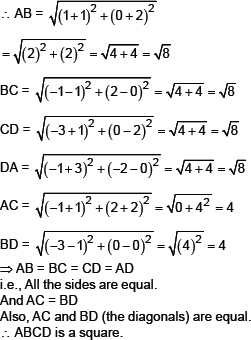(ii) Let the points be A (�3, 5), B(3, 1), C(0, 3) and D(�1, �4).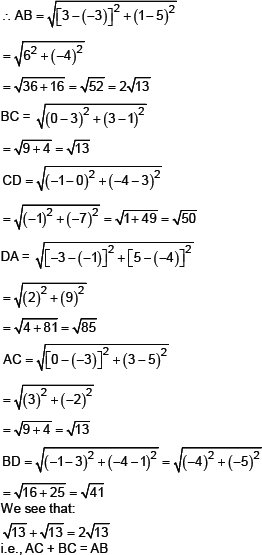⇒ A, B, C and D are collinear. Thus, ABCD is not a quadrilateral.
(iii) Let the points be A (4, 5), B (7, 6), C (4, 3) and D(1, 2).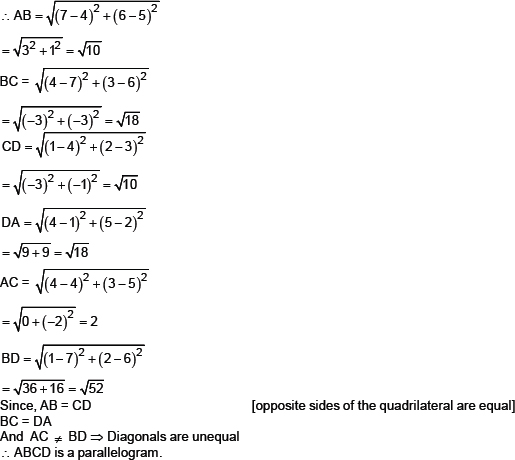Q.7.   Find the point on the x-axis which is equidistant from (2, �5) and (�2, 9).
Sol. We know that any point on x-axis has its ordinate = 0.
Let the required point be P(x, 0).
Let the given points be A(2, �5) and B(�2, 9)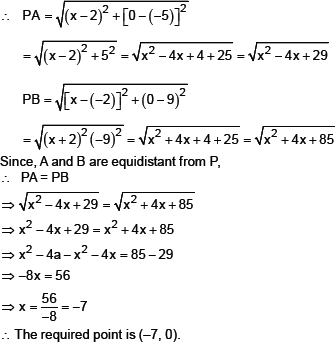Q.8.   Find the values of y for which the distance between the points P(2, �3) and Q(10, y) is 10 units.
Sol. The given points are P(2, �3) and Q(10, y).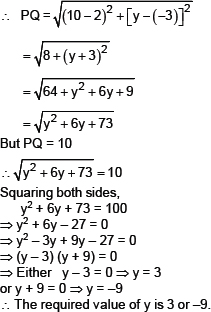Q.9.   If Q (0, 1) is equidistant from P(5, �3) and R(x, 6), find the values of x. Also find the distances QR and PR.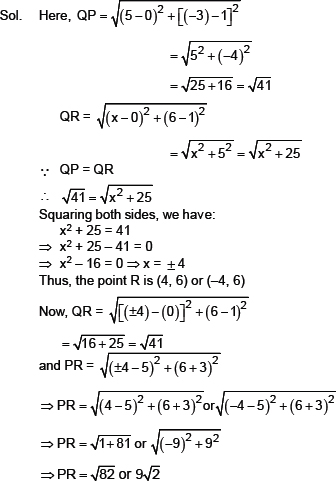Q.10.   Find a relation between x and y such that the point (x, y) is equidistant from the point (3, 6) and (�3, 4).
Sol. Let the points be A(x, y), B (3, 6) and C(�3, 4).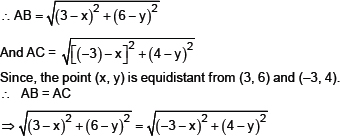Squaring both sides,
(3 – x)2 + (6 – y)2 = (–3 – x)2 + (4 – y)2
⇒ (9 + x2 – 6x) + (36 + y2 – 12y) = (9 + x2 + 6x) + (16 + y2 – 8y)
⇒ 9 + x2 – 6x) + 36 + y2 – 12y – 9 – x2 – 6x – 16 – y2 + 8y
⇒ –6x – 6x + 36 – 12y – 16 + 8y = 0
⇒ –12x – 4y + 20 = 0
⇒ –3x – y + 5 = 0
⇒ 3x + y – 5 = 0
which is the required relation between x and y.
Exercise 7.2
Q.1.   Find the coordinates of the point which divides the join of (�V1, 7) and (4, �V3) in the ratio 2 : 3.
Sol.     Let the required point be P (x, y).
Here, the end points are:
(–1, 7) and (4, –3)
∵Ratio = 2 : 3 = m1 : m2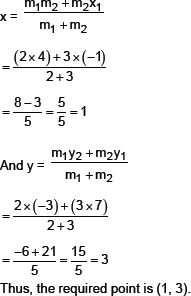Q.2.   Find the coordinates of the points of trisection of the line segment joining (4, –1) and (–2, –3).
Sol.     Let the given points be A(4, –1) and B(–2, –3).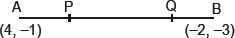Let the poi ts P and Q trisect AB.
i.e., AP = PQ = QB
i.e., P divides AB in the ratio of 1 : 2
Q divides AB in the ratio of 2 : 1
Let the coordinates fo P be (x, y).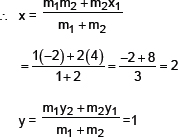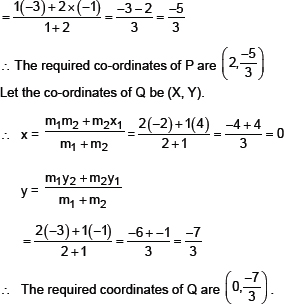Q.3.   To conduct Sports Day activities, in your rectangular shaped school ground ABCD, lines have been drawn with chalk powder at a distance of 1 m each. 100 flower pots have been placed at a distance of 1 m from each other along AD, as shown in the figure. Niharika runs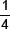th the distance AD on the 2nd line and posts a green flag. Preet runs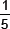th the distance AD on the eighth line and posts a red flag. What is the distance between both the flags? If Rashmi has to post a blue flag exactly halfway between the line segment joining the two flags, where should she post her flag?
Sol.     Let us consider ‘A‘ as origin, then AB is the x-axis. AD is the y-axis.
Now, the position of green flag-post is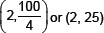And the position of red flag-post is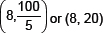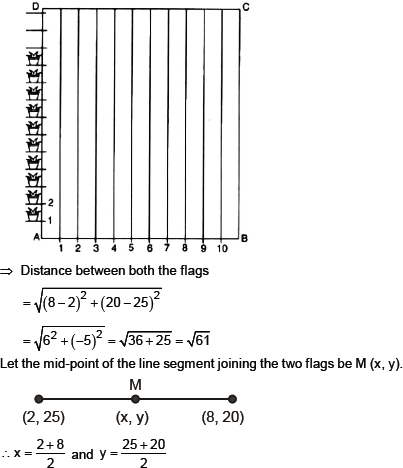or x = 5 and y = (22.5).
Thus, the blue flag is on the 5th line at a distance 22.5 m above AB.
Q.4.   Find the ratio in which the line segment joining the points (–3, 10) and (6, –8) is divided by (–1, 6).
Sol.     Let the given points are: A (–3, 10) and B (6, –8).
Let the point P (–1, 6) divides AB in the ratio m1 : m2.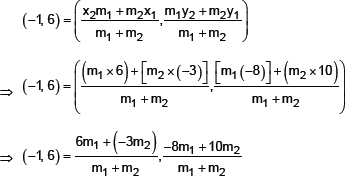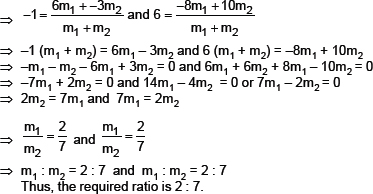Q.5.   Find the ratio in which the line segment joining A (1, –5) and B (–4, 5) is divided by the x-axis. Also find the coordinates of the point of division.
Sol.     The given points are: A (1, –5) and B (–4, 5).
Let the required ratio = k : 1 and the required point be P (x, y).
Part-I: To find the ratio
Since the point P lies on x-axis,
∴ Its y-coordinates is 0.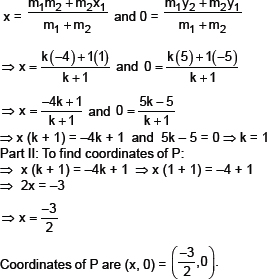Q.6.   If (1, 2) (4, y), (x, 6) and (3, 5) are the vertices of a parallelogram taken in order, find x and y.
Sol.     We have the parallelogram vertices
A (1, 2), B (4, y), C (x, 6) and D (3, 5)
Since, the diagonals of a parallelogram bisect each other.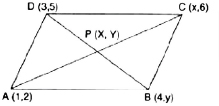∴ The coordinates of P are: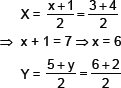⇒; 5 + y = 8 T y = 3
∴ The required values of x and y are:
x = 6, y = 3
Q.7.   Find the coordinates of a point A, where AB is the diameter of a circle whose centre is (2, –3) and B is (1, 4).
Sol.     Here, centre of the circle is O (2, –3).
Let the end points of the diameter be
A (X, Y) and B (1, 4)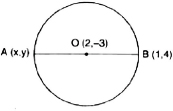The centre of a circle bisects the diameter.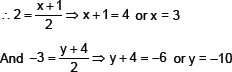Hence the coordinates of A are (3, –10).
Q.8.   If A and B are (–2, –2) and (2, –4), respectively, find the coordiantes of P such that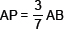and P lies on teh line segment AB.
Sol.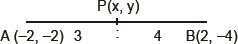Here, the given points are
A(–2, –2) and B (2, –4)
Let the coordinates of P are (x, y).
Since, the point P divides AB such that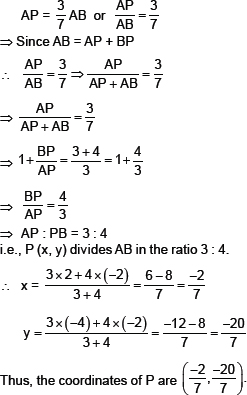Q.9.   Find the coordinates of the points which divide the line segment joining A(–2, 2) and B (2, 8) into four equal parts.
Sol.     Here, the given points are:
A(–2, 2) and B (2, 8)
Let P1, P2 and P3 divide AB in four equal parts.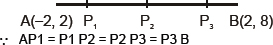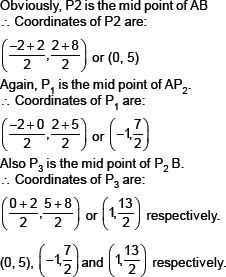Q.9.   Find the area of a rhombus if its vertices are (3, 0), (4, 5), (–1, 4) and (–2, –1) taken in order.
Sol.     Let the vertices of the given rhombsu are:
A (3, 0), B (4, 5), C (–1, 4) and D (–2, –1)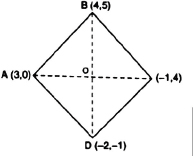∵AC and BD are the diagonals of rhombus ABCD.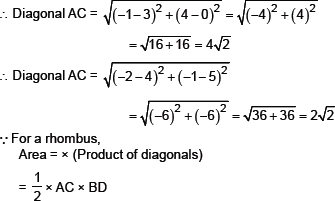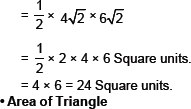I. If A (x1, y1); B (x2, y2) and C (x3, y3) are the vertices of ΔABC, then the area of ΔABC =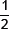[x1 (y2 y3) + x2 (y3 – y1) + x3 (y1 – y2)].
II. The three points A, B and C are collinear if and only if area of ΔABC = 0.
Exercise 7.3
Q.1.   Find the area of the triangle whose vertices are:
(i) (2, 3), (–1, 0), (2, –4)                                                (ii) (–5, –1), (3, –5), (5, 2)
Sol.     (i) Let the vertices of the triangle be
A (2, 3), B (–1, 0) and C(2, –4)
Here, x1 = 2, y1 = 3
x2 = –1, y2 = 0
x3 = 2, y3 = –4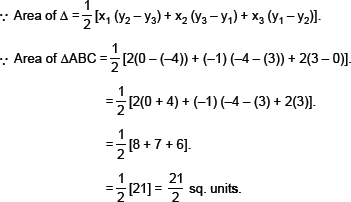(ii) Let the vertices of the triangle be
A(–5, –1), B(3, –5) and C(5, 2)
i.e., x1 = –5, y1 = –1
x2 = 3, y2 = –5
x3 = 5, y3 = 2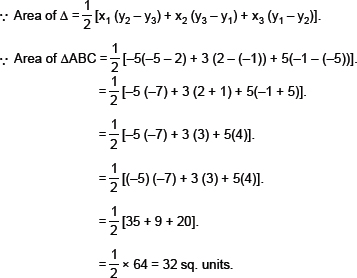Q.2.   In each of the following find the value of ‘k’, for which the points are collinear.
(i) (7, –2), (5, 1), (3, k)                                                (ii) (8, 1), (k, –4), (2, –5)
Sol.     The given three points will be collinear if the Δ Formed by them has zero area.
(i) Let A (7, –2), B (5, 1) and C(3, k) be the vertics of a triangle.
∴ The given points will be collinear, if are (ΔABC) = 0
or 7(1 – k) + 5 (k + 2) + 3(–2 –1) = 0
⇒ 7 – 7k + 5k + 10 + (–6) – 3 = 0
⇒ 7 – 9 + 5k – 7k = 0
⇒ 8 – 2k = 0
⇒ 2k = 0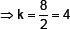The required value of k = 4.
(ii) Let (8, 1), (k, –4) and (2, –5) be the verticles of a triangle.
∴ For the above points being collinear, ar (ΔABC) = 0
i.e., 8 (–4 + 5) + k (–5 –1) + 2[1 – (–4)] = 0
⇒ 8 (+1) + k (–6) + 2(5) = 0
⇒ 8 + (–6k) + 10 = 0
⇒ –6k + 18 = 0
⇒ k = (–18) + (–6) = 3
Thus, k = 3.
Q.3.   Find the area of the triangel formed by joining the mid-points of the sides of the triangle whose vertices are (0, –1), (2, 1) and (0, 3). Find the ratio of this area to the area of the given triangle.
Sol.     Let the vertices of the triangle be A(0, –1), B(2, 1) and C(0, 3).
Let D, E and F be the mid-points of the sides BC, CA and AB respectively. Then: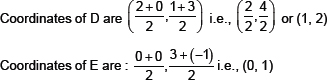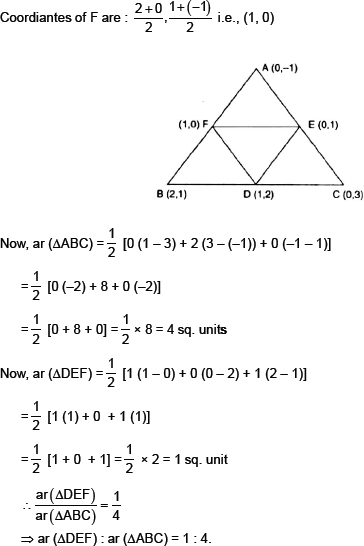Q.4.   Find the area of the quadrilateral whose vertices, taken in order, are (–4, –2), (–3, –5), (3, –2) and (2, 3).
Sol.     Let A (–4, –2), B (–3, –5), C(3, –2) and D(2, 3) be the vertices of the quadrilateral.
Let us join diagonal BD.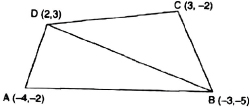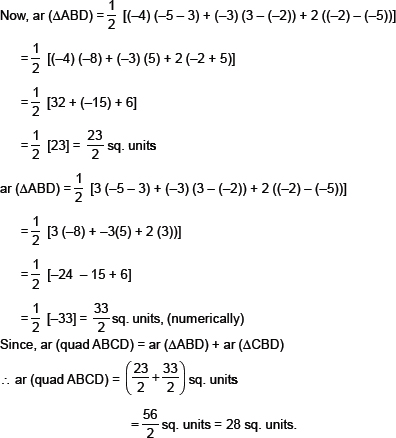Q.5.   You have studies in class IX (Chapter 9, Example–3) that, a median of a triangle divides it into two triangles of equal areas. Verify this result for ��ABC whose vertics are A(4, –6), B(3, –2) and C(5, 2).
Sol.     Here, the vertices of the triangle are A(4, –6), B(3, –2) and C(5, 2).
Let D be the mid-point of BC.
∴ The coordiantes of the mid point D are: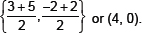Since AD divides the triangle ABC into two parts i.e., ΔABD and ΔACD,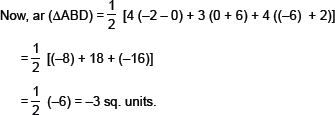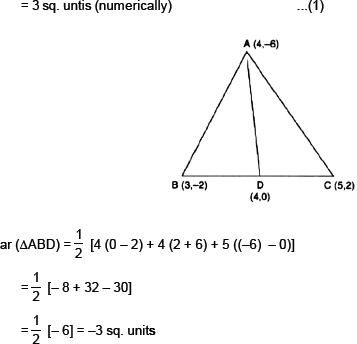= 3 sq. units (numerically)                                                  ...(2)
From (1) and (2)
ar (ΔABD) = ar (ΔACD))
i.e. A median divides the triangle into two triangles of equal areas.
Exercise 7.4
Q.1.   Determine the ratio in which the line 2x + y – 4 = 0 divides the line segment joining the points A(2, –20 and B (3, 7).
Sol.     Let the required ratio be k : 1 and the point C divides them in the abvoe ratio.
∴ Coordinates of C are: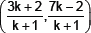Since the point C lies on the given line 2x + y – 4 = 0,
∴ We have: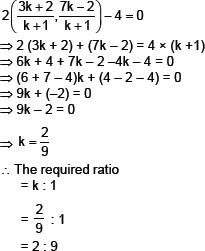Q.2.   Find a relation between x and y if the points (x, y), (1, 2) and (7, 0) are collinear.
Sol.     The given points are:
A (x, y), B (1, 2) and C(7, 0)
The points A, B and C will be collinear if
x (2 – 0) + 1 (0 – y) + 7 (y – 2) = 0
or if 2x – y + 7y – 14 = 0
or if 2x + 6y – 14 = 0
or if x + 3y – 7 = 0
which is the require relation between x and y.
Q.3.   Find the centre of a circle passing through the points (6, –6), (3, –7) and (3, 3).
Sol.     Let P (x, y) be the center of the circle passing through
A(6, –6), B(3, –7) and C(3, 3).
∴ AP = BP = CP
Taking AP = BP, we have AP2 = BP2
⇒ (x – 6)2 + (y + 6)2 = (x – 3)2 + (y + 7)2
⇒ x2 – 12x + 36 + y2 + 12y + 36 = x2 – 6x + 9 + y2 + 14y + 49
⇒ –12x + 6x + 12y – 14y + 72 – 58 = 0
⇒ –6x – 2y + 14 = 0
Taking BP = CP, we have BP2 = CP2
⇒ (x – 3)2 + (y + 7)2 = (x – 3)2 + (y – 3)2
⇒ x2 – 6x + 9 + y2 + 14y + 49 = x2 – 6x + 9 + y2 – 6y + 9
⇒ –6x + 6x + 14y + 6y + 58 – 18 = 0
⇒ 20y + 40 = 0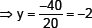From (1) and (2),
3x – 2 – 7 = 0
⇒ 3x = 9 ⇒ x = 3
i.e., x = 3 and y = –2
∴ The required centre is (3, –2).
Q.4.   The two opposite vertics of a square are (–1, 2) and (3, 2). Find the coordiantes of the other two vertices.
Sol.     Let us have a square ABCD such that A(–1, 2) and C(3, 2) are the opposite vertices. Let B(x, y) be an unknown vertex. Since all sides of a square are equal,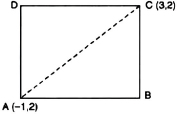∴ AB = BC
⇒ AB2 = BC2
⇒ (x + 1)2 + (y – 2)2 = (x – 3)2 + (y – 2)2
⇒ 2x + 1 = –6x + 9
⇒ 8x = 8 ⇒ x = 1
...(1)
Since each angle of a square = 90°,
∴ABC is a right angled triangle.
∴Using Pythagoras theorem, we have:
AB2 + BC2 = AC2
⇒ (x + 1)2 + (y – 2)2 + (x – 3)2 + (y – 2)2
= [(3 + 1)2 + (2 – 2)2]
⇒ 2x2 + 2y2 + 2x – 4y – 6x – 4y + 1 + 4 + 9 + 4 = 16
⇒ 2x2 + 2y2 – 4x – 8y + 2 = 0
⇒ x2 + y2 – 2x – 4y + 1 = 0
...(2)
Substituting the value of x from (1) into (2) we have:
1 + y2 – 2 – 4y + 1 = 0
⇒ y2 – 4y + 2 – 2 = 0
⇒ y2 – 4y = 0
⇒ y(y – 4) = 0
⇒ y = 0 or y = 4
Hence, the requried other two vertices are: (1, 0) and (1, 4).
Q.5.   The Class X students of a secondary school in Krishinager have bee allotted a rectangular plot of land for their gardening activity. Saplins of Gulmohar are planted on the boundary at a distance of 1 m from each other. There is a triangular grassy lawn in the plot as shown in the Fig. The students are to sow seeds of flowering plants on the remaining area of the plot.
(i) Taking A as origin, find the coordinates of the vertices of the triangle.
(ii) What will be the coordinates of the vertices of ∴PQR if C is the origin?
Also calculate the areas of the triangles in these cases. What do you observe?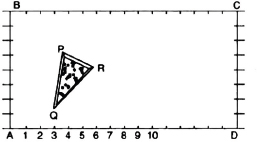Sol.     (i) By taking A as the origin and AD and AB as the coordinate axes, we have P(4, 6), Q(3, 2) and R(6, 5) as the vertices of ∴PQR.
(ii) By taking C as the origin and CB and CD as the coordinate axes, then the vertices of ΔPQR are
P (12, 2), Q (13, 6) and R(10, 3)
Now, ar (ΔPQR)
[wnen P(4, 6), Q(3, 2) and R(6, 5) are the vertices]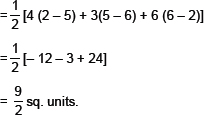Thus, in both cases, the area of ΔPQR is the same.
Q.6.   The vertices of a DABC are A (4, 6), B (1, 5) and C(7, 2). A line is drawn to intersect sides AB and AC at D and E respectively, such that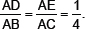Calculate the area of the ΔADE and compare it with the area of ΔABC. (Recall Theorem 6.2 and Theorem 6.6).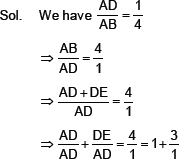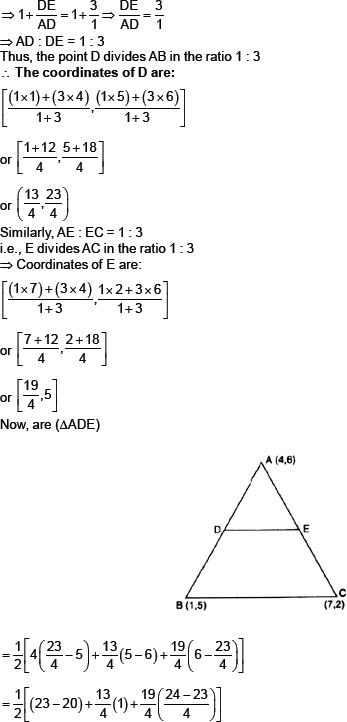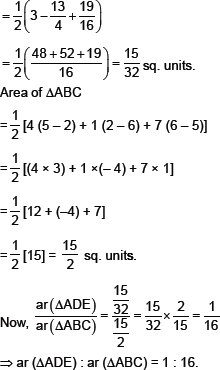Q.7.   Let A (4, 2), B(6, 5) and C(1, 4) be the vertices of ΔABC.
(i) The median from A meets BC at D. Find the coordinates of the point D.
(ii) Find the coordinates of the point P on AD such that AP : PD = 2 : 1.
(iii) Find the coordinates of points Q and R on medians Be and CF respectively such that BQ : QE
= 2 : 1 and CR : RF = 2 : 1.
(iv)What do you observe?
(v) If A (x1, y1), B(x2 , y2) and C(x3 , y3) are the vertices of ΔABC, find the coordiantes of the centroid of the triangle.
Sol.     We have the vertices of ΔABC as A(4, 2) B(6, 5) and C(1, 4).
(i) Since AD is a median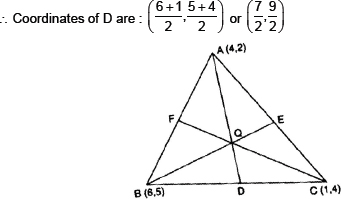(iv)What do you observe?
(ii) Since AP : PD = 2 : 1 i.e., P divides AD in the ratio 2 : 1.
∴ Coordinates of P are: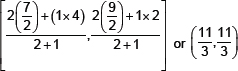(iv)What do you observe?
(iii) BQ : QE = 2 : 1 ⇒ [The point Q divides BE in the radio 2 : 1]
∴ Coordinates of Q are: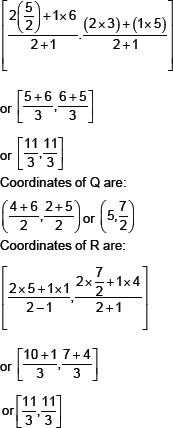(iv)We observe that P, Q and R represent the same point.
(v) Here, we have A(xx, yy), B(xx, yy), C(xx, yy) as the vertices of ��ABC. Also AD, BE and CF are its medians.
∴D, E and F are the mid points of BC, CA and AB respectively.
We know, the centroid is a point on a median, dividing it in the ratio 2 : 1.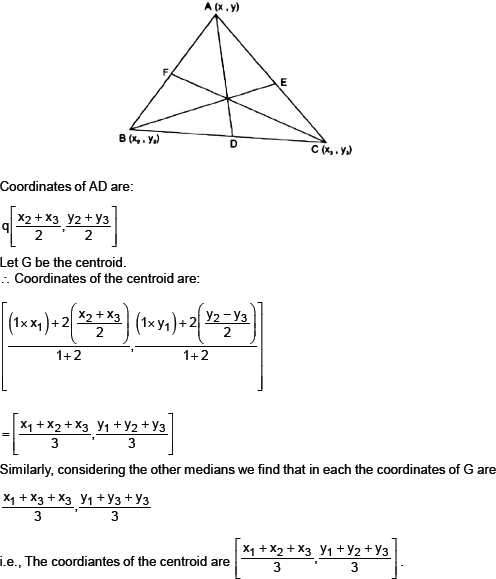Q.8.   ABCD is a rectangle formed by the points A(–1, –1), B (–1, 4), C (5, 4) and D(5, –1).
P, Q, R and S are the mid points of AB, BC, CD and DA respectively. Is the quadrilateral PQRS a square? a rectangle? or a rhombus? Justify your answer.
Sol.     We have a rectangle whose vertices are A(–1, –1), B (–1, 4), C(5, 4) and D(5, –1).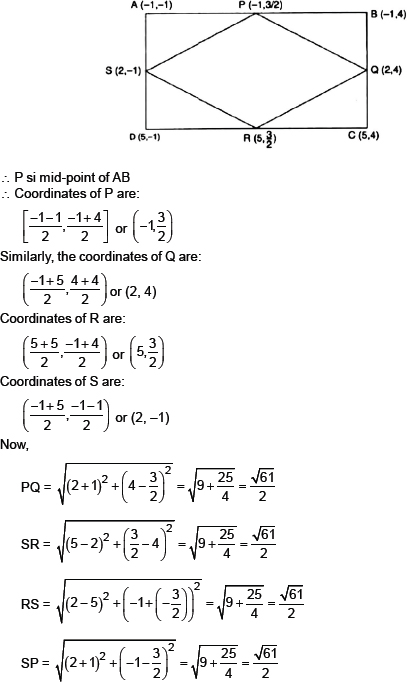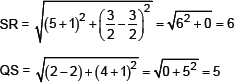We see that:
PQ = QR = RS = SP
i.e., all sides of PQRS are equal.
∴ It can be a square or a rhombus.
But its diagonals are not equal.
i.e., PR ≠ QS
∴ PQRS is a rhombus.